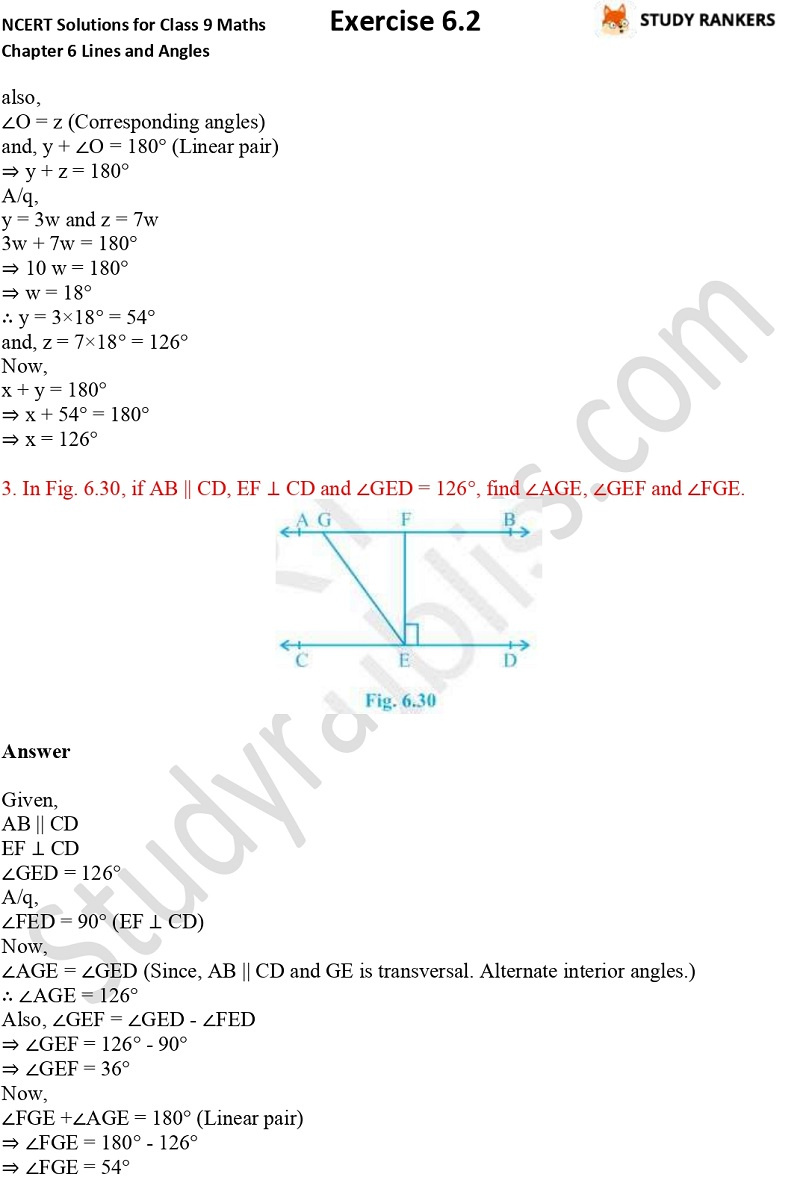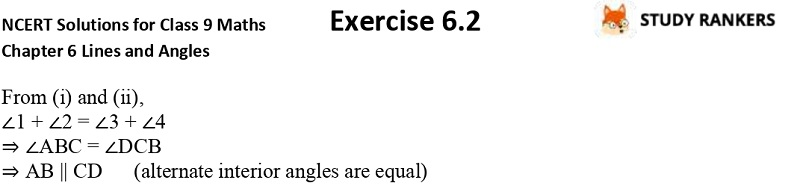>

## NCERT Solutions for Class 9 Maths Chapter 6 Lines and Angles Exercise 6.2

If you want NCERT Solutions of Chapter 6 Lines and Angles Exercise 6.2 then you can find them here. Class 9 Maths NCERT Solutions can be helpful if you want to improve your marks in the examinations. These NCERT questions and answers are prepared by Studyrankers experts that are detailed and accurate so you can easily understand them. It will surely help you exploring important formulas and revising the basic concepts provided in the chapter.

Ex 6.2 has total 6 questions which are about finding the values of x and y in given figures and proving a given statement etc.X